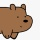Home
IT Knowledge
Inspiration
Languages
EN

# Python - literate through list

0 points
Created by:Palus-Bear
586

In this article, we would like to show you how to iterate through the list in Python.

## 1. Using for loop

In this example, we use simple for loop to iterate through the `my_list` and print its items.

``````my_list = ['A', 'B', 'C']

for x in my_list:
print(x)``````

Output:

``````A
B
C``````

## 2. Using while loop

To iterate through the list using while loop, we need to:

• specify the iterator - in our case we set `i = 0`,
• use `len()` function to specify the length of the list.
• increment the iterator (`i`) after each iteration.

Practical example:

``````my_list = ['A', 'B', 'C']

i = 0
while i < len(my_list):
print(my_list[i])
i = i + 1``````

Output:

``````A
B
C``````